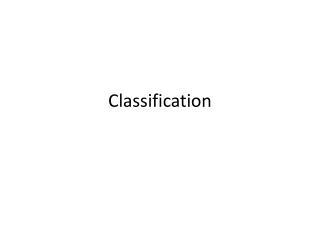DownloadDownload PresentationClassification

Classification

Télécharger la présentationClassification

- - - - - - - - - - - - - - - - - - - - - - - - - - - E N D - - - - - - - - - - - - - - - - - - - - - - - - - - -
Presentation Transcript

1. Classification

2. Introduction • A discriminant is a function that separates the examples of different classes. For example • IF (income > Q1 and saving >Q2) • Then low risk customer • ELSE • High risk customer • Having a rule like this the main application is prediction. Once we have a rule that fits the past data, then we can make correct prediction for new instances.

3. Introduction • Given a new customer application with a certain income and savings we can easily decide whether it is low risk or high risk. • In some cases, instead of making a 0/1 (low risk/ high risk) type decision, we may want to calculate a probability namely P(Y|X) where X are the customer attributes and Y is 0 or 1 respectively for low risk and high risk. • From this perspective we can see classification as learning an association from X to Y.

4. Introduction • Then for a given X=x, if we have P(Y=1|X=x) = 0.8 we say that the customer has an 80% probability of being high risk or equivalently a 20% probability of being low risk. • y = g(x/Q) where g(.) is the model and Q are it parameters. • Remember “y” is a number in regression and “y” is a class code (e.g 0/1) in case of classification. • g(.) is the regression function in regression and in classification, it is the discriminant function separating the instances of different classes.

5. Example • An AI program optimizes the parameters Q such that the approximation error is minimized, that is, our estimates are as closes as possible to the correct values given in the training set. • Example we observe customer’s yearly income and savings, which we represented by two random variable X1 and X2. • The credibility of the customer is denoted by a bernoulli random variable C conditioned on the observables X=[X1, X2] where C=1 indicates a high risk customer and C=0 indicates low risk customer.

6. Example • If we know P(C|X1,X2) when a new application arrives with X1= x1 and X2 = x2, we can choose • C=1 if P(C=1|x1,x2) > 0.5 • C=0 otherwise OR • C=1 if P(C=1|x1,x2) > P(C=0|x1,x2) • C=0 otherwise

7. Bayes’ rule • P(C|X) = P(X|C) P(C) / P(X) • P(C|X) is called Posterior • P(X|C) is called Likelihood • P(C) is called Prior • P(X) is called Evidence • P(C=1) is called prior probability that C takes the value 1, which in our example corresponds to the probability that a customer is high risk, regardless of the value X

8. Bayes’ rule • It is called prior probability because it is the knowledge we have as to the value of C before looking at the observation X, satisfying • P(C=0) + P(C=1) = 1 • P(X|C) is called the class likelihood and is the conditional probability that an event belonging to C has the associated observation values X. • P(x1,x2| C=1) is the probability that a high risk customer has his or her X1= x1 and X2 = x2. • It is what the data tell us regarding the class.

9. Bayes’ rule • P(X) the evidence, is the marginal probability that an observation X seen regardless of whether it is a +ve or –ve example. • P(X) = P(X|C=1).P(C=1) + P(X|C=0).P(C=0) • Because of normalization by the evidence the posterior sum up to 1. • P(C=0|X) + P(C=1|X) = 1

10. General Case • In the general case, we have K mutually exclusive and exhaustive classes. Ci, i=1,2…k. • We have prior probabilities satisfying P(Ci)>= 0 and • P(X|Ci) is the probability of seeing X as the input when it is known that it belongs to class Ci. • The posterior probability of Class Ci can be calculated as P(Ci|X) = P(X|Ci) P(Ci) / P(X) • P(X) = and for minimum error the Bayes’ classifier choose the class with the highest posterior probability that is we choose Ci if P(Ci|X)=maxk(P(Ck|X)

11. Learning Multiple Classes • In the general case, we have K classes as Ci, i=1,2…K and an input instance belongs to one and exactly one of them. • The training set is now of the form x={xt,rt}tN where r has k dimensions and rt = { 1 if xtεCi} • In AI for classification we would like to learn the boundary separating the instances of one class from the instances of all other classes. • We can view a k-class classification problem as k two-class problems.